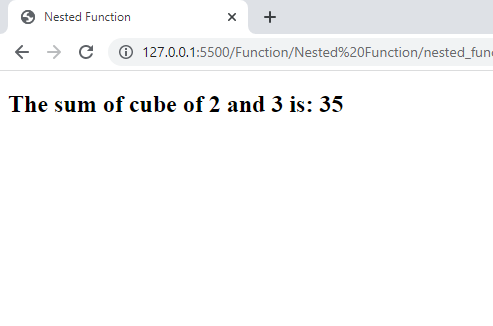# Nested Function In JavaScript

in STEMGeeks2 years agoImage Source

Nested function, as the name implies, is the function that contains another function inside it. In JavaScript, you can nest a function inside another function. The nested function is usually referred to as the inner function and the function where another function is nested is known as outer or parent function. The nested function can access the variable/parameter and the other functions defined in the outer function whereas the outer function can't access the variable/parameter and the function defined inside the inner function. And in order to make your function work, you just need to call the outer function.

Lets suppose we want to add cube of two numbers. For this we will make use of nested function. The outer function will find the sum of cube of certain number and the nested function will just find the cube. So what I am saying here is the inner function will be responsible for finding the cube and the outer function is responsible for finding the sum of cube of those number.

``````<!DOCTYPE html>
<html lang="en">
<title>Nested Function</title>
<script>
{
function findCube(z)
{
return z*z*z;
}
return findCube(x) + findCube(y);
}